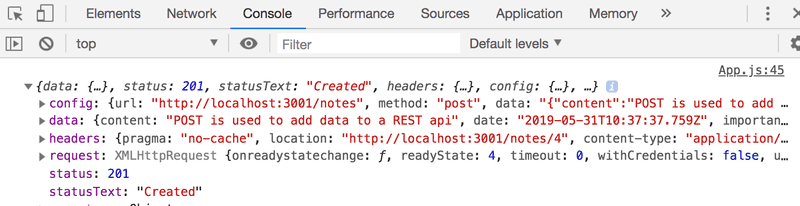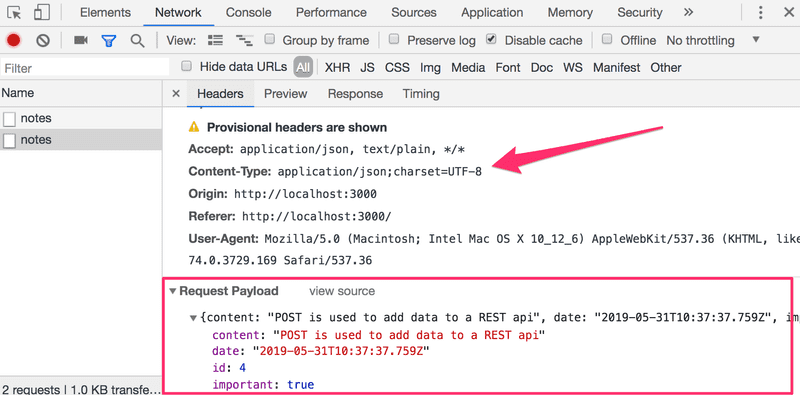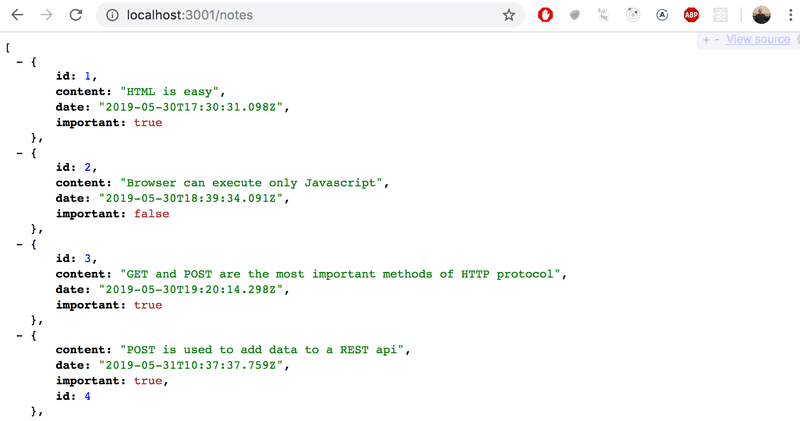d

Get a full fake REST API with zero coding in less than 30 seconds (seriously) 在不到30秒(严肃地)的情况下得到一个完整的模拟 REST API，0编码

Json-server 与 REST API 的教科书定义提供的描述不完全匹配，但是自称是 RESTful 的大多数服务都不完全匹配。

### REST

Json-server 要求以 JSON 格式发送所有数据。 实际上，这意味着数据必须是格式正确的字符串，并且请求必须包含值为application/jsonContent-Type 请求头。

### Sending Data to the Server

【发送数据到服务器】

``````addNote = event => {
event.preventDefault()
const noteObject = {
content: newNote,
date: new Date(),
important: Math.random() < 0.5,
}

axios    .post('http://localhost:3001/notes', noteObject)    .then(response => {      console.log(response)    })}````````````addNote = event => {
event.preventDefault()
const noteObject = {
content: newNote,
date: new Date(),
important: Math.random() > 0.5,
}

axios
.post('http://localhost:3001/notes', noteObject)
.then(response => {
setNotes(notes.concat(response.data))      setNewNote('')    })
}``````### Changing the importance of notes

【改变便笺的重要性】

``````const Note = ({ note, toggleImportance }) => {
const label = note.important
? 'make not important' : 'make important'

return (
<li>
{note.content}
<button onClick={toggleImportance}>{label}</button>
</li>
)
}``````

App组件定义了 toggleImportanceOf事件处理函数的初始版本，并将其传递给每个Note组件:

``````const App = () => {
const [notes, setNotes] = useState([])
const [newNote, setNewNote] = useState('')
const [showAll, setShowAll] = useState(true)

// ...

const toggleImportanceOf = (id) => {    console.log('importance of ' + id + ' needs to be toggled')  }
// ...

return (
<div>
<h1>Notes</h1>
<div>
<button onClick={() => setShowAll(!showAll)}>
show {showAll ? 'important' : 'all' }
</button>
</div>
<ul>
{notesToShow.map((note, i) =>
<Note
key={i}
note={note}
toggleImportance={() => toggleImportanceOf(note.id)}          />
)}
</ul>
// ...
</div>
)
}``````

``() => { console.log('importance of 3 needs to be toggled') }``

``console.log('importance of ' + id + ' needs to be toggled')``

``console.log(`importance of \${id} needs to be toggled`)``

``````const toggleImportanceOf = id => {
const url = `http://localhost:3001/notes/\${id}`
const note = notes.find(n => n.id === id)
const changedNote = { ...note, important: !note.important }

axios.put(url, changedNote).then(response => {
setNotes(notes.map(note => note.id !== id ? note : response.data))
})
}``````

``const changedNote = { ...note, important: !note.important }``

``````const note = notes.find(n => n.id === id)
note.important = !note.important

axios.put(url, note).then(response => {
// ...``````

``````axios.put(url, changedNote).then(response => {
setNotes(notes.map(note => note.id !== id ? note : response.data))
})``````

``notes.map(note => note.id !== id ? note : response.data)``

Map 方法通过将旧数组中的每个项映射到新数组中的一个项来创建一个新数组。 在我们的示例中，新数组被有条件地创建，即如果note.id !== id为true，我们只需将项从旧数组复制到新数组中。 如果条件为 false，则将服务器返回的 note 对象添加到数组中。

### Extracting communication with the backend into a separate module

【将与后端的通信提取到单独的模块中】

``````import axios from 'axios'
const baseUrl = 'http://localhost:3001/notes'

const getAll = () => {
return axios.get(baseUrl)
}

const create = newObject => {
return axios.post(baseUrl, newObject)
}

const update = (id, newObject) => {
return axios.put(`\${baseUrl}/\${id}`, newObject)
}

export default {
getAll: getAll,
create: create,
update: update
}``````

App 组件使用 import访问模块:

``````import noteService from './services/notes'
const App = () => {``````

``````const App = () => {
// ...

useEffect(() => {
noteService      .getAll()      .then(response => {        setNotes(response.data)      })  }, [])

const toggleImportanceOf = id => {
const note = notes.find(n => n.id === id)
const changedNote = { ...note, important: !note.important }

noteService      .update(id, changedNote)      .then(response => {        setNotes(notes.map(note => note.id !== id ? note : response.data))      })  }

const addNote = (event) => {
event.preventDefault()
const noteObject = {
content: newNote,
date: new Date().toISOString(),
important: Math.random() > 0.5
}

noteService      .create(noteObject)      .then(response => {        setNotes(notes.concat(response.data))        setNewNote('')      })  }

// ...
}

export default App``````

``````noteService
.getAll()
.then(response => {
setNotes(response.data)
})``````

App 组件只使用response对象的 response.data 属性。

``````noteService
.getAll()
.then(initialNotes => {
setNotes(initialNotes)
})``````

``````import axios from 'axios'
const baseUrl = 'http://localhost:3001/notes'

const getAll = () => {
const request = axios.get(baseUrl)
return request.then(response => response.data)
}

const create = newObject => {
const request = axios.post(baseUrl, newObject)
return request.then(response => response.data)
}

const update = (id, newObject) => {
const request = axios.put(`\${baseUrl}/\${id}`, newObject)
return request.then(response => response.data)
}

export default {
getAll: getAll,
create: create,
update: update
}``````

``````const getAll = () => {
const request = axios.get(baseUrl)
return request.then(response => response.data)
}``````

``````const getAll = () => {
const request = axios.get(baseUrl)
return request.then(response => {    return response.data  })}``````

``````const App = () => {
// ...

useEffect(() => {
noteService
.getAll()
.then(initialNotes => {        setNotes(initialNotes)      })
}, [])

const toggleImportanceOf = id => {
const note = notes.find(n => n.id === id)
const changedNote = { ...note, important: !note.important }

noteService
.update(id, changedNote)
.then(returnedNote => {        setNotes(notes.map(note => note.id !== id ? note : returnedNote))      })
}

const addNote = (event) => {
event.preventDefault()
const noteObject = {
content: newNote,
date: new Date().toISOString(),
important: Math.random() > 0.5
}

noteService
.create(noteObject)
.then(returnedNote => {        setNotes(notes.concat(returnedNote))        setNewNote('')
})
}

// ...
}``````

You do not know JS 一书中，对这个议题进行了很好的解释well，但是解释有很多页

### Cleaner syntax for defining object literals

【用于定义对象字面量的更清晰的语法】

``````import axios from 'axios'
const baseUrl = 'http://localhost:3001/notes'

const getAll = () => {
const request = axios.get(baseUrl)
return request.then(response => response.data)
}

const create = newObject => {
const request = axios.post(baseUrl, newObject)
return request.then(response => response.data)
}

const update = (id, newObject) => {
const request = axios.put(`\${baseUrl}/\${id}`, newObject)
return request.then(response => response.data)
}

export default {
getAll: getAll,
create: create,
update: update
}``````

``````{
getAll: getAll,
create: create,
update: update
}``````

``````{
getAll,
create,
update
}``````

``````import axios from 'axios'
const baseUrl = 'http://localhost:3001/notes'

const getAll = () => {
const request = axios.get(baseUrl)
return request.then(response => response.data)
}

const create = newObject => {
const request = axios.post(baseUrl, newObject)
return request.then(response => response.data)
}

const update = (id, newObject) => {
const request = axios.put(`\${baseUrl}/\${id}`, newObject)
return request.then(response => response.data)
}

export default { getAll, create, update }``````

``````const name = 'Leevi'
const age = 0``````

``````const person = {
name: name,
age: age
}``````

``const person = { name, age }``

### Promises and errors

【承诺和错误】

``````const getAll = () => {
const request = axios.get(baseUrl)
const nonExisting = {
id: 10000,
content: 'This note is not saved to server',
date: '2019-05-30T17:30:31.098Z',
important: true,
}
return request.then(response => response.data.concat(nonExisting))
}````````````axios
.get('http://example.com/probably_will_fail')
.then(response => {
console.log('success!')
})
.catch(error => {
console.log('fail')
})``````

catch 方法通常是通过将其置于Promise链的更深处来使用的。

``````axios
.put(`\${baseUrl}/\${id}`, newObject)
.then(response => response.data)
.then(changedNote => {
// ...
})``````

catch 方法可用于在承诺链的末尾定义一个处理程序函数，一旦承诺链中的任何承诺抛出错误，承诺就变成rejected，就会调用该函数。

``````axios
.put(`\${baseUrl}/\${id}`, newObject)
.then(response => response.data)
.then(changedNote => {
// ...
})
.catch(error => {
console.log('fail')
})``````

``````const toggleImportanceOf = id => {
const note = notes.find(n => n.id === id)
const changedNote = { ...note, important: !note.important }

noteService
.update(id, changedNote).then(returnedNote => {
setNotes(notes.map(note => note.id !== id ? note : returnedNote))
})
.catch(error => {      alert(        `the note '\${note.content}' was already deleted from server`      )      setNotes(notes.filter(n => n.id !== id))    })}``````

``notes.filter(n => n.id !== id)``